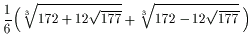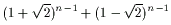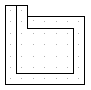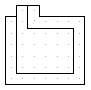Polystrips

On this page I present results of my first attempt to count polystrips.
For simplicity I don't rotate or reflect pieces. The number of polystrips in all positions T(n) is given in the table below.

We can count two subsets of polystrips and two supersets :

Two-directional polystripsThe number of 2dir polystrips is
L2(n) = 2n - 2

Three-directional polystripsThe number of 3dir polystrips is
L3(n) = c1 x1n  + c2 x2n  + c3 x3n - 2n
where xi are roots of  x3-2x2-1=0
x1=2.20556943040059
x2= -0.10278471520030 + 0.66545695115281 i
x3= -0.10278471520030 - 0.66545695115281 i
and
c1=1.26798014703815
c2=0.36600992648092 + 0.54201687825554 i
c3=0.36600992648092 - 0.54201687825554 i

The asymptotic behavior is

 lim L3(n)/L3(n-1) = x1 = 2.20556...=Paths on square gridTheir number is
U0(n)=2 3n-2

Paths on square grid without sharp U-turnsTheir number is

 U1(n)=Number of polystrips

L2(n)<=L3(n)<=T(n)<=U1(n)<=U0(n)

If the following limit exists then

 2.205569... <= lim T(n)/T(n-1) <= 2.414214...

Similar estimates was found for polyminoes [Klarner's constant]

 3.91336 <= lim A(n)/A(n-1) <= 4.649551

 psc T(n) T(n)/T(n-1) L2(n) L3(n) T(n) U1(n) U0(n) 2 1 2 2 2 2 2 2 3 2 6 3.000 6 6 6 6 6 4 3 14 2.333 14 14 14 14 18 5 7 34 2.429 30 34 34 34 54 6 13 82 2.412 62 82 82 82 162 7 30 194 2.366 126 194 194 198 486 8 65 462 2.381 254 454 462 478 1458 9 153 1094 2.368 510 1054 1094 1154 4374 10 346 2590 2.367 1022 2430 2590 2786 13122 11 818 6110 2.356 2046 5570 6110 6726 39366 12 1893 14422 2.360 4094 12706 14422 16238 118098 13 4313 33938 2.353 8190 28866 33938 39202 354294 14 10067 79910 2.3545 16382 65350 79910 94642 1062882 15 23621 187674 2.3485 32766 147502 187674 228486 3188646 16 55313 441038 2.3500 17 129647 1034142 2.3447 18 303720 2426326 2.3462 19 711078 5681662 2.3416 20 1665037 13312246 2.3430 21 3894282 31137950 2.3390 22 9111343 72871914 2.3402 23 21290577 170287162 2.3368 24 49770844 398122698 2.3379 25 116206114 929561314 2.3348 26 271435025 2171377246 2.3359 27

My questions:

1. What is the asymptotic ratio of number of polyominoes with holes and without holes?
2. What is the asymptotic ratio of perimeter and area of polyominoes?
3. Polystrip and polytrees with n>=9 can't form rectangle. (The cave in the piece below can't be filled). Polyominoes with n>=22 can't form rectangle (There are more caves than pieces of corresponding shape). Is it possible to find similar examples with smaler  polyominoes?